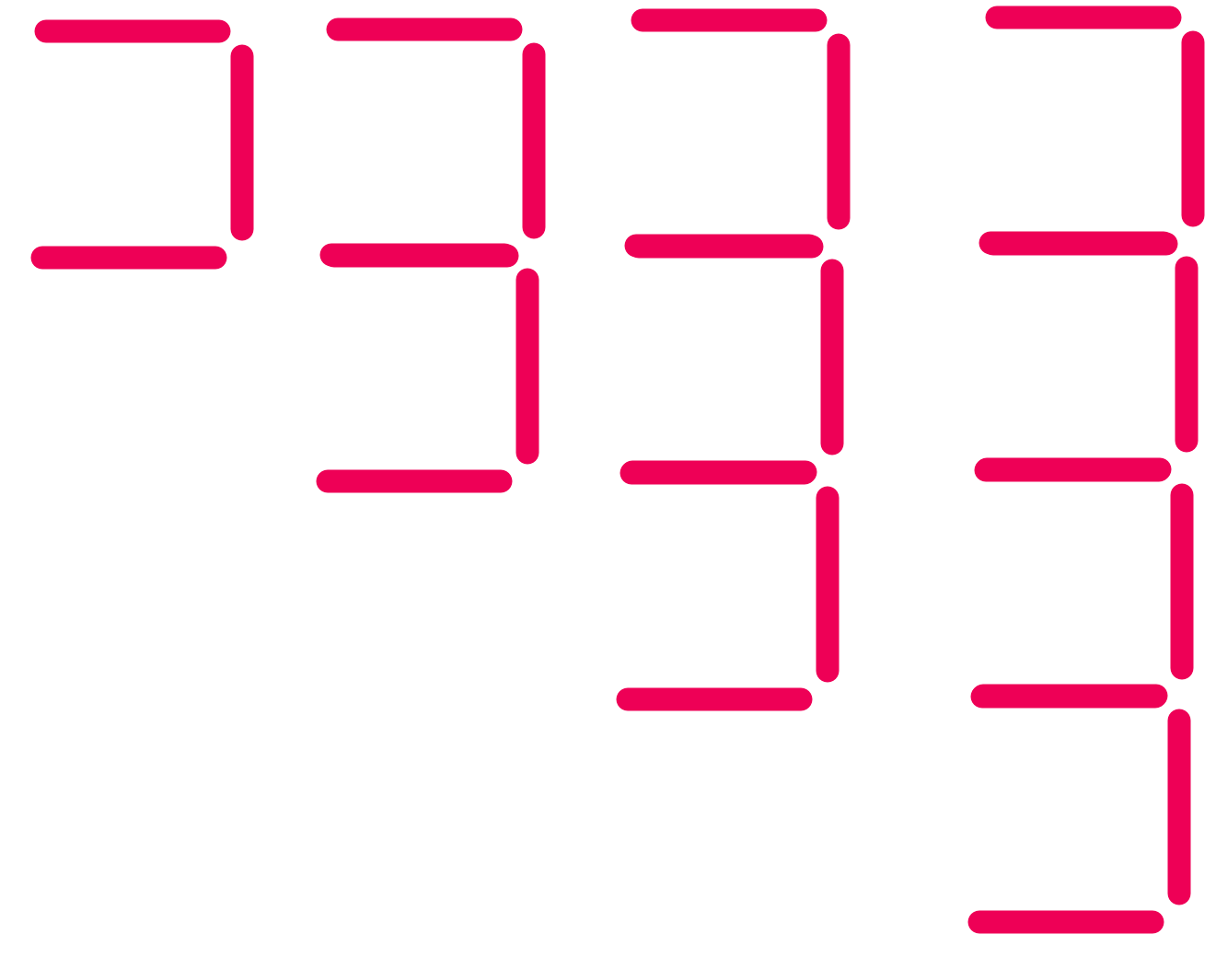Generating Terms

# Generating Terms

Basic, GCSE(F),

A sequence is a list of numbers, or a pattern. The numbers, or patterns, are related to each other by a rule. The next number or pattern in the list can be worked out from the previous number or pattern using the rule.

Each number in the list is called a term. The first number is the first term, the second number is the second term, and so on.

The rule that links the terms is called the term-to-term rule.

To work out the next term in a sequence, it is often necessary to work out what the rule is. Compare two terms, and determine how the second rule has changed from the first.

Check: After a term-to-term rule has been worked out, always compare it against another pair of terms to make sure that it is correct.

## Examples

1. A term-to-term rule is given as multiply the last term by 2 and subtract 3. What are the next two terms in the sequence, if the first term is 5?

The second term is given by 5 x 2 - 3 = 7

The third term is 7 x 2 - 3 = 11.

2. A sequence is made up from a set of lines. How many lines will there be in the 5th and 6th terms of the sequence?